If a small amount of antimony is added to germanium crystal

(a) the antimony becomes an acceptor atom

(b) there will be more free electrons than holes in the semiconductor

(c) its resistance is increased

(d) it becomes a p-type semiconductor

Concept Questions :-

Types of semiconductors
High Yielding Test Series + Question Bank - NEET 2020

Difficulty Level:

In forward biasing of the p-n junction

(a) the positive terminal of the battery is connected to n-side and the depletion region becomes thin

(b) the positive terminal of the battery is connected to n-side and the depletion region becomes thick

(c) the positive terminal of the battery is connected to p-side and the depletion region becomes thin

(d) the positive terminal of the battery is connected to p-side and the depletion region becomes thick

Concept Questions :-

PN junction
High Yielding Test Series + Question Bank - NEET 2020

Difficulty Level:

A transistor is operated in common emitter configuration at  such that a change in the base current from  to  produces a change in the collector current from  to . The current gain is

1. 75                                               2. 100

3. 25                                                4. 50

Concept Questions :-

Transistor
High Yielding Test Series + Question Bank - NEET 2020

Difficulty Level:

In the following figure, the diodes which are forward biased, are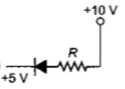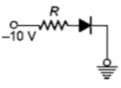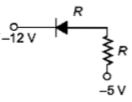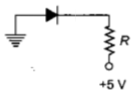(1) C and D only

(2) A and C only

(3) B only

(4) Band D only

Concept Questions :-

PN junction
High Yielding Test Series + Question Bank - NEET 2020

Difficulty Level:

Pure Si at 500 K has equal number of electron $\left({n}_{\mathit{e}}\right)$ and hole $\left({n}_{\mathit{h}}\right)$ concentrations of $1.5×{10}^{16}{\mathrm{m}}^{-3}.$ Doping by indium increases ${n}_{\mathit{h}}$ to  The doped semiconductor is of

(a) n-type with electron concentration

(b) p-type with electron concentration

(c) n-type with electron concentration

(d) p-type with electron concentration

Concept Questions :-

Types of semiconductors
High Yielding Test Series + Question Bank - NEET 2020

Difficulty Level:

A Zener diode, having breakdown voltage equal to 15 V, is used in a voltage regulator circuit shown in the figure. The current through the diode is :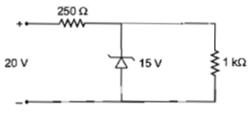1. 10 mA                                                    2. 15 mA

3. 20 mA                                                     4. 5 mA

Concept Questions :-

Applications of PN junction
High Yielding Test Series + Question Bank - NEET 2020

Difficulty Level:

Which one of the following statement is false?

(a) Pure Si doped with trivalent impurities gives a p-type semiconductor

(b) Majority carries in a n-type semiconductor are holes

(c) Minority carries in a p-type semiconductor are electrons

(d) The resistance of intrinsic semiconductor decreases with increase of temperature

Concept Questions :-

Types of semiconductors
High Yielding Test Series + Question Bank - NEET 2020

Difficulty Level:

The device that can act as a complete electronic circuit is

1. Junction diode

2. Integrated circuit

3. Junction transistor

4. Zener diode

Concept Questions :-

Transistor
High Yielding Test Series + Question Bank - NEET 2020

Difficulty Level:

A common emitter amplifier has a voltage gain of 50, an input impedance of 100$\mathrm{\Omega }$ and an output impedance of 200$\mathrm{\Omega }$. The power gain of the amplifier is-

1. 500

2. 1000

3. 1250

4. 50

Concept Questions :-

Applications of Transistor
High Yielding Test Series + Question Bank - NEET 2020

Difficulty Level:

The following figure shows a logic gate circuit with two inputs A and B and the output Y. The voltage waveforms of A, B and Y are as given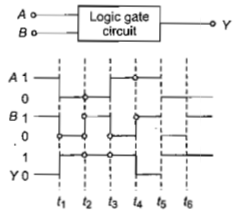The logic gate is

(a) $\mathrm{NOR}$ gate                                                 (b) $\mathrm{OR}$ gate

(c)  gate                                                 (d) gate

Concept Questions :-

Logic gates